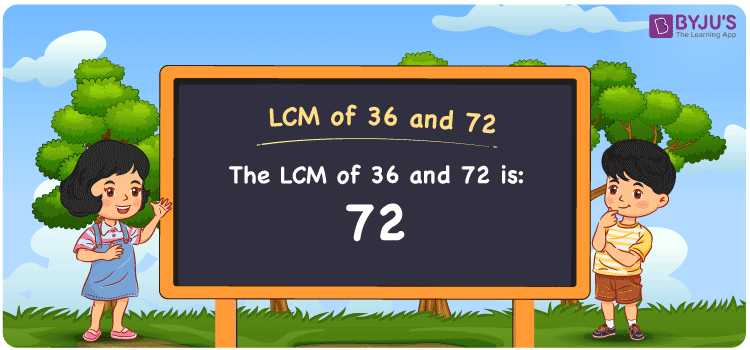Checkout JEE MAINS 2022 Question Paper Analysis : Checkout JEE MAINS 2022 Question Paper Analysis :

# LCM of 36 and 72

LCM of 36 and 72 is 72. The common multiple divisible evenly by the numbers 36 and 72 is the LCM. Students can learn to get the least common multiples of 36 and 72 from the common multiples. (36, 72, 108, 144, 180, 216, 252, ….) and (72, 144, 216, 288, 360, 432, ….) are the multiples of 36 and 72. The LCM of two numbers can be found using methods like listing the multiples, prime factorization and division method.

## What is LCM of 36 and 72?

The answer to this question is 72. The LCM of 36 and 72 using various methods is shown in this article for your reference. The LCM of two non-zero integers, 36 and 72, is the smallest positive integer 72 which is divisible by both 36 and 72 with no remainder.## How to Find LCM of 36 and 72?

LCM of 36 and 72 can be found using three methods:

• Prime Factorisation
• Division method
• Listing the multiples

### LCM of 36 and 72 Using Prime Factorisation Method

The prime factorisation of 36 and 72, respectively, is given by:

36 = 2 x 2 x 3 x 3 = 2² x 3²

72 = 2 x 2 x 2 x 3 x 3 = 2³ x 3²

LCM (36, 72) = 72

### LCM of 36 and 72 Using Division Method

We’ll divide the numbers (36, 72) by their prime factors to get the LCM of 36 and 72 using the division method (preferably common). The LCM of 36 and 72 is calculated by multiplying these divisors.

 2 36 72 2 18 36 2 9 18 3 9 9 3 3 3 x 1 1

No further division can be done.

Hence, LCM (36, 72) = 72

### LCM of 36 and 72 Using Listing the Multiples

To calculate the LCM of 36 and 72 by listing out the common multiples, list the multiples as shown below

 Multiples of 36 Multiples of 72 36 72 72 144 108 216 144 –

LCM (36, 72) = 72

## Video Lesson on Applications of LCM## LCM of 36 and 72 Solved Examples

Find the smallest number exactly divisible by 36 and 72.

Solution:

We know that

LCM is the smallest number exactly divisible by 36 and 72.

Multiples of 36 = 36, 72, 108, 144, ….

Multiples of 72 = 72, 144, 216, ….

Hence, the LCM of 36 and 72 is 72.

## Frequently Asked Questions on LCM of 36 and 72

### How to determine the LCM of 36 and 72?

The LCM of 36 and 72 can be found using the methods below

Division method

Prime Factorisation

Listing the multiples

### With the help of the prime factorisation method, determine the LCM of 36 and 72.

To get the LCM, the factors should be known

36 = 2 x 2 x 3 x 3 = 2² x 3²

72 = 2 x 2 x 2 x 3 x 3 = 2³ x 3²

LCM (36, 72) = 72

### What is the GCF if the LCM of 36 and 72 is 72?

LCM x GCF = 36 x 72

As the LCM = 72

72 x GCF = 2592

GCF = 2592/72 = 36